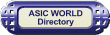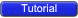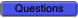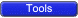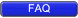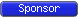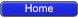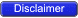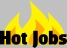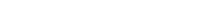Sample Verilog Questions asked in Interviews. Please contribute with your questions. If you are looking for answers please refer to website Site FAQ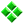Differentiate between Inter assignment Delay and Inertial Delay.What are the different State machine Styles ? Which is better ? Explain disadvantages and advantages.What is the difference between the following lines of code ? reg1<= #10 reg2 ; reg3 = # 10 reg4 ;What is the value of Var1 after the following assignment ?reg Var1; initial begin Var1<= "-" endIn the below code, Assume that this statement models a flop with async reset. In this, how does the synthesis tool, figure out which is clock and which is reset. Is the statements within the always block is necessary to find out this or not ?``` 1 module which_clock (x,y,q,d); 2 input x,y,d; 3 output q; 4 reg q; 5 6 always @ (posedge x or posedge y) 7 if (x) 8 q <= 1'b0; 9 else 10 q <= d; 11 12 endmodule ``` You could download file which_clock.v hereWhat is the output of the two codes below ?``` 1 module quest_for_out(); 2 3 integer i; 4 reg clk; 5 6 initial begin 7 clk = 0; 8 #4 \$finish; 9 end 10 11 always #1 clk = ! clk; 12 13 always @ (posedge clk) 14 begin : FOR_OUT 15 for (i=0; i < 8; i = i + 1) begin 16 if (i == 5) begin 17 disable FOR_OUT; 18 end 19 \$display ("Current i : %g",i); 20 end 21 end 22 endmodule ``` You could download file quest_for_out.v here``` 1 module quest_for_in(); 2 3 integer i; 4 reg clk; 5 6 initial begin 7 clk = 0; 8 #4 \$finish; 9 end 10 11 always #1 clk = ! clk; 12 13 always @ (posedge clk) 14 begin 15 for (i=0; i < 8; i = i + 1) begin : FOR_IN 16 if (i == 5) begin 17 disable FOR_IN; 18 end 19 \$display ("Current i : %g",i); 20 end 21 end 22 endmodule ``` You could download file quest_for_in.v hereWhy cannot initial statement be synthesizeable ?Consider a 2:1 mux; what will the output F be if the Select (sel) is "X" ?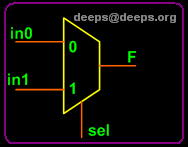What is the difference between blocking and nonblocking assignments ?What is the difference between wire and reg data type ?Write code for async reset D-Flip-Flop.Write code for 2:1 MUX using different coding methods.Write code for a parallel encoder and a priority encoder.What is the difference between === and == ?What is defparam used for ?What is the difference between unary and logical operators ?What is the difference between tasks and functions ?What is the difference between transport and inertial delays ?What is the difference between casex and case statements ?What is the difference between \$monitor and \$display ?What is the difference between compiled, interpreted, event based and cycle based simulators ?What is code coverage and what are the different types of code coverage that one does ?